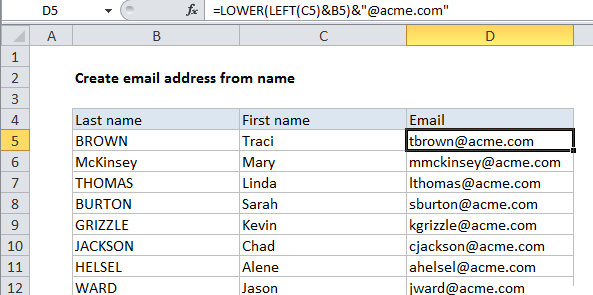## Excel Office

Excel How Tos, Tutorials, Tips & Tricks, Shortcuts

# How to create email address from name in Excel

To build email addresses using first and last names, you can use a formula that concatenates values, with help from the LOWER and LEFT functions as needed. See example below:

## Formula

`=LOWER(LEFT(first)&last)&"@domain.com"`## Explanation

In the example shown, the formula in D5 is:

`=LOWER(LEFT(C5)&B5)&"@"&"acme.com"`

### How this formula works

For a name like “Tim Brown”, this formula builds an email address like “[email protected]”.

Also See:   Remove file extension from filename in Excel

First, the LEFT function is used to get the first letter from the first name in column C. Usually, the LEFT function gets a “num_chars” value for the second argument, but the argument is optional and defaults to 1 if omitted.

`LEFT(C5) // get first character from first name`

The first letter of the first name is then joined to the last name using the concatenation operator (&), and the result is wrapped in the LOWER function, which forces all text to lower case.

`LOWER(LEFT(C5)&B5) // lower case`

Finally, result is jointed again to “@” and then to the domain. The domain is kept separate for convenience only. The formula could be written like this:

Also See:   LEFT, LEFTB functions: Description, Usage, Syntax, Examples and Explanation

### With a named range

To simply the formula, you could create a named range “domain” to hold the domain name, then rewrite the formula like this:

`=LOWER(LEFT(first)&last)&domain`

Changing the value in the named range will then update all email addresses at once.

1.